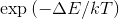This is a 2D Ising model simulation. It uses Metropolis criterion, where a magnetic domain is always flipped when ΔE<0, otherwise with probability. Domain oriented "up" is orange, "down" is white. Domains are selected one by one, each with probability (1-"randomness"). Metropolis criterion is applied only to selected domains, so that randomness=0 applies metropolis criterion to every domain, which may result in artificial, oscillation-like behaviour. Randomness close to one slows down the algorithm. Additional parameter Fr, responsible for friction, can be added to ΔE. Coupling constant J describes interaction between domains. Buttons on the bottom can be used to control simulation speed or reset it. I used terra.js. Here is the simulation code.

Author: Łukasz Mioduszewski

Magnetization per domain m=0

Total magnetization M=0

Coupling J=0.1

Temperature T=0.1

External field h=0

Friction Fr=0

Randomness:
Coupling:
Temperature:
External field:
Friction: